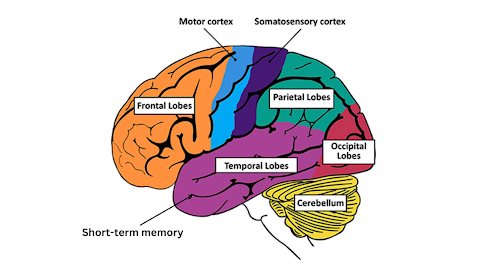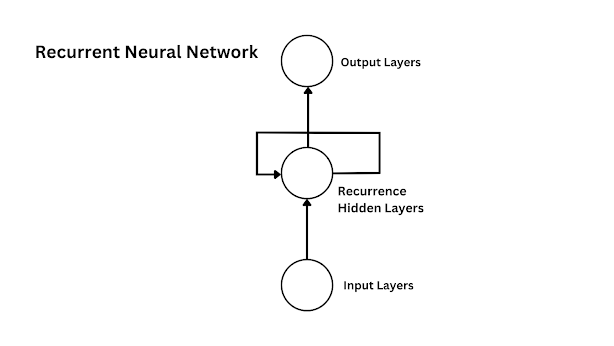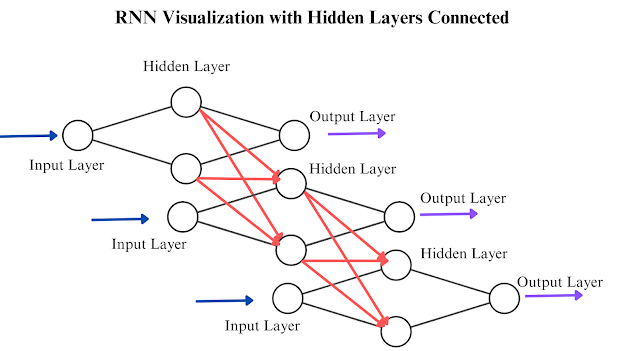# Recurrent Neural Networks: An Overview With Python Example

In this article, we are going discuss what basically Recurrent Neural Networks actually do, and why are they so special, We'll look ath Python Example

## Introduction

You often heard about AI learning to trade, predicting stock prices, recognizing speech, translating language, and even generating human-level text from scratch in a modern scenario. All the advancements in these areas started from the idea of sequence modeling. Sequence modeling is a method of understanding and predicting patterns within sequence-based data like stock prices for example. The idea started from a core concept of creating a machine learning model which can learn and predict sequence data. One such early candidate for sequence modeling which became so much popular for time series datasets is the Recurrent Neural Networks or RNNs. A Recurrent Neural Network is a type of Neural Network Architecture specifically devoted to tasks involving sequences of data or we can call time series datasets. In this article, we are going discuss what basically Recurrent Neural Networks actually do, and why are they so special, we also give some Python examples to understand RNNs work in the real world. So let's get started!

## The Basic Intuition

Most of us know that the idea of deep learning has a great inspiration from biology specifically, the human brain. Recurrent Neural Networks are inspired by a part of the brain called the Temporal Lobe which resides in short-term memory. Short-term memory is the part of the brain that helps us to remember what happened around us recently. In other words, it stores information temporarily. This part contributes a lot to our ability to handle languages, understand patterns, and understand what is going around us recently.Source: NIH

RNNs are created based on a similar idea where the network can capture short-term dependencies in the given dataset. This doesn't mean that RNNs are exact copies of short-term memory in our brains, but RNNs are Artificial Neural Network structures for handling sequential temporary information that can be used for different tasks like Langauge Modelling, Speech Recognition, Machine Translation, and more.

## The Idea of Recurrence

Let's start with the traditional Feed Forward Neural Network. The structure of basic Feed Forward Neural Networks consists of an input layer, multiple hidden layers, and an output layer. Information is passed through these layers to produce the corresponding outputs. But if you think about it, these Feed Forward Neural Networks are not really good for sequence-based data. This is because each data point in the sequence datasets has some kind of relation to the previous data points. For example, the sentence "Jim is a young intelligent boy" and "Amy is a young intelligent girl". In these two sentences, you can observe that the last word "boy/girl" depends on what comes first in the sentence. In the case of text data, the relationships between words in the sequence are important for understanding the context and meaning of the sentences. This is also applicable to all time series datasets.

Even though Feed Forward Neural Networks can capture the patterns in datasets, They will not be good at time series. So to handle time series, we need something called Recurrence. So, what is Recurrence? Recurrence is the most fundamental idea behind Recurrent Neural Networks and other species of RNNs like LSTM (Long Short Term Memory) and GRU (Gated Recurrent Units) where you take multiple Feed Forward Neural Networks, connect the hidden layers together to form a sequential loop and you'll get a Recurrent Neural Network. By doing this, a Recurrence is formed inside the network. In such a case, the data coming to the network is also processed based on the weights in the recurrent unit that stores the information about the previous data the network saw instead of directly passing it to the next layer.RNN Diagram

This understanding is quite good when we are just starting with RNNs, Maybe you see this figure shown above as a structure of RNN in most tutorials. But the real beauty of RNNs can be identified by visualizing them in our brains. Think of RNNs as a collection of Feed Forward Neural Networks where the hidden layers are connected together one by one. Let's consider a really simple FFNN with one input layer, 2 hidden layers, and one output layer and see how we can create an RNN,RNN Visualization

What can we understand from the image? we can see that the hidden layers of three FFNNs are connected in such a way similar to how the hidden layers are connected to the output layer in traditional Neural Networks. Now challenge your brain by thinking a ton of these Feed Forward Neural Networks with different neuron sizes where the hidden layer is connected one by one. You'll see a forest of Neural Networks! This is what a Recurrent Neural Network is.

So if you take the case of the second Feed Forward Neural Network from the image, its prediction is based on the input from the input layer and the inputs coming from the recurrent hidden layers. This mean RNNs were able to recall what happened in the previous step which is important for predicting time series datasets.

Alright, one more important thing, each of these single Feed Forward Neural Networks where the hidden layers are connected is called a Time step. So from the above image, there are three timesteps.

## The Structure of Recurrent Neural Networks

We have discovered an effective method for conceptualizing RNNs in our minds, but when it comes to the mathematical aspect, it is necessary to use a diagrammatic way to represent them. Things are simple, imagine you are looking from the top of this network structure above, what you'll see looks something like this,

This is the top view of the previous image we have seen, Understand that each of these circles are layers or neurons and each of the lines are the connections between each of the neurons. Since you are watching from the top, you'll not see all the neurons and connections. Anyway, this is a good diagrammatical representation of Recurrent Neural Networks. If we represent it in this way, why can't we create multiple structures from it? What I mean is that you can create multiple types of RNNs by changing the structure of the above diagram. So Recurrent Neural Networks are of different types,

### One-to-One (Single Time step)

One-to-One RNNs are basically regular Feed Forward Neural Networks. It takes an individual input and produces an individual output without any sequence context. It's like giving the network a standalone piece of information, and it doesn't involve sequences or time steps. For example, you could use this for tasks like image classification, where each image is treated independently.

### One-to-Many

In this setup, you provide a single input to the RNN, but it generates a sequence of outputs in response. You can find this model in action when comes to image captioning: you give the RNN an image, and it generates a sequence of words that describe the contents of the image.

### Many-to-One

Here, you input a sequence of data to the RNN, and it produces a single output at the end of the sequence. For instance, in sentiment analysis, you could input a sentence, and the RNN predicts whether the sentiment of the sentence is positive, negative, or neutral.

### Many-to-Many (Same Length)

This structure involves inputting a sequence of data and getting a corresponding sequence of outputs, where both input and output sequences have the same length. Machine translation is a classic example: you provide a sentence in one language, and the RNN produces a sequence of words representing the translation in another language.

### Many-to-Many (Different Lengths)

This scenario occurs when you need to input a sequence and produce an output sequence, but the lengths of the input and output sequences differ. Speech recognition is a fitting example: you input an audio recording of varying lengths, and the RNN outputs a sequence of recognized words, which might not match the length of the input.

## How Does Recurrent Neural Network Work?

Now, you might have a good way of visualizing RNNs, but how do they manage the sequential data, and what actually they are doing? To start, let's understand what RNNs do in the case of feeding a few words,

Let's assume this is a trained RNN, and what happens when we fed text information to it.

The sentence as you guessed is "How are you?". The first word "How" is converted to a vector and fed into the first input layer of the network. Since we assume the RNN is trained, we'll get the next word as output which is "are". This prediction is based on what word has the highest probability to come next in the sequence.

Then the word "are" is fed as input to the second time step and predicts "you". Now, here's the interesting part: in the second step, the RNN uses both the current input ("are") and also some hidden information from the step before. This helps the RNN remember what came before and make better predictions. This is how RNNs extract information from the previous sequence.

As we move to the third step, the RNN uses the current input ("you") and combines it with information from not only the step just before but also the one before that. This gives the fancy "?" symbol that comes after every Question. The RNN keeps getting better at using previous words to understand and predict what comes next in the sequence.

This is also applicable to other forms of sequential data like stock prices, audio signals, etc.

So how are the computations done? In the case of Feed Forward Neural Network, the computation is simple as,

$\hat{y} = \phi{(W_{ij}X_{j} + b_j)}$

where, $$W_{ij}$$ is the weights, $$X_{j}$$ is the inputs, $$b_j$$ is the bias term, and $$\phi$$ is the activation function.

Since RNNs also need to deal with different time steps because of the connections between hidden layers through time, the computations of weights and inputs are a little different, To understand, let's mark some weights in the diagram,

The $$W_{xh}$$ are the weights connecting from the input layer to the hidden layer of each time step. The $$W_{hh}$$ are the weights in the hidden layer, and finally, the $$W_{oh}$$ are the weights corresponding to the output layer.

The $$X_1$$, $$X_2$$, and, $$X_3$$ are the inputs to each corresponding time step, and $$y_1$$, $$y_2$$, $$y_3$$ are the outputs from each timestep. However, we need to represent the information passing from one time step to another. Let's call this $$h_{t}$$ as for the hidden state in a particular time step.

A lot of gibberish in the diagram, but, if you look closely, the $$h_{t}$$, $$h_{t-1}$$, and, $$h_{t-2}$$ are the hidden states in the hidden layers of each time step $$t$$, in our case, there are only three-time steps.

Now intuitively, what do you think the formula to calculate $$h_{t}$$ when considering the last time step?, is it simply $$W_{hh}.X_t$$ ? Partially yes, but we need to also consider $$h_{t-1}$$ coming from the hidden layer of the previous time step which is simply $$W_{hh}.h_{t-1}$$. So the whole general formula can be computed as,

$h_t = \phi{(W_{xh}. X_t + W_{hh}.h_{t-1} + b_{t})}$

This is the formula to calculate hidden states in each time step no matter how large the RNN is. What's important is that we need to also consider the previous time step as input in the current time step.

Usually, when comes to RNN, we use the Tanh (Hyperbolic tangent) activation function in the hidden units of each time step. This is because Tanh activation maps inputs between -1 and +1 which helps to manage issues like the vanishing gradient problem, which we'll discuss in the upcoming article. There are some other advantages of using Tanh activation, like capturing both positive and negative information.

$h_t = tanh(W_{xh}. X_t + W_{hh}.h_{t-1} + b_{t})$

So what bout the output $$y_t$$? To compute $$y_t$$, let's zoom in on what happens in the output layers,

Typically, in RNNs, $$h_{t}$$ is passed in both directions including the hidden layers of different time steps and the output layers. So when computing the output $$y_t$$ comes from RNN, we also consider hidden states, so the output $$y_t$$ in general can be computed as,

$y_t = \phi{(W_{oh}.h_t + b_{o})}$

The use of the activation function in the output layer depends on what problem you are trying to solve, for instance, if you are solving a probability-based problem you can use sigmoid or softmax.

To wrap up, information is passed through a Recurrent Neural Network (RNN) in a series of time steps. Each time step remembers the information extracted from the previous time steps so that it can be propagated throughout the entire network.

## Recurrent Neural Network Example With Python & TensorFlow

Alright, let's create a simple Vanilla Recurrent Neural Network using TensorFlow, but before that, let's create a random sequential dataset for testing our RNN, here is the code for creating a simple sequential dataset,

import numpy as np# Parametersnum_samples = 10sequence_length = 4# Generate a synthetic time series datasetdef generate_sequence(start_value, length):    sequence = [start_value]    for _ in range(length - 1):        next_value = sequence[-1] + np.random.normal(0, 0.1)        sequence.append(next_value)    return sequence# Create the datasetX = []y = []for _ in range(num_samples):    start_value = np.random.uniform(-1, 1)    sequence = generate_sequence(start_value, sequence_length)    target_value = sequence[-1] + np.random.normal(0, 0.1)    X.append(sequence[:-1])  # Input sequence    y.append(target_value)    # Target value to predictX = np.array(X)y = np.array(y)print(X.shape)print(y.shape)

This code creates a random dataset of specified "num_samples" and "sequence_length". Now let's create an RNN using TensorFlow,

import tensorflow as tfmodel = tf.keras.Sequential([    tf.keras.layers.SimpleRNN(units=64, activation='tanh', input_shape=(None, 1)),    tf.keras.layers.Dense(units=1)])model.compile(loss='mean_squared_error', optimizer='adam')model.fit(X, y, epochs=50)

Here we are using the Mean Squared Error (MSE) because this is basically a regression problem where we need to predict the next sequence after each sequence in the input data. You can evaluate the model's performance by the following code,

model.evaluate(X, y)-------0.004521036054939032

Remember this is a really simplified version of RNN which can learn sequential information, Now try If you put the "num_samples" to 1000 or 2000, you'll see that the MSE increasing, Why? There are two problems that might occur. One is when the datasets are not so sequential in nature, and the second might be the vanishing gradient problem. The plain Vanilla architecture of RNNs has limitations due to the vanishing and exploding gradient problems. To overcome this limitation, we introduced the more efficient versions of RNNs like LSTM (Long Short Term Memory) Networks and GRU (Gated Recurrent Units).

We'll discuss more about these in the upcoming articles. Understand that this article discusses the whole concept behind the information passing forward through a Recurrent Neural Network, but in order for RNNs to learn we need to train it using an algorithm called Backpropagation Through Time (BPTT) which needs an entire article.

## Conclusion

In conclusion, this article has provided a comprehensive introduction to Recurrent Neural Networks (RNNs) that not only familiarizes us with their fundamental concepts but also offers a visual approach to understanding their intricate architecture. By delving into the visualization of RNNs, we've gained valuable insights into how these networks process sequential data, allowing us to appreciate their significance in various fields. Moreover, the article has explored different types of RNNs. We have looked at a simple Python Example with TensorFlow to create a simple RNN.